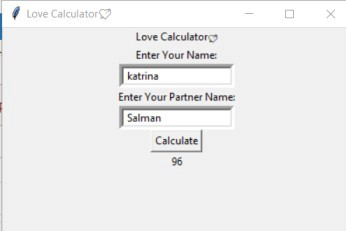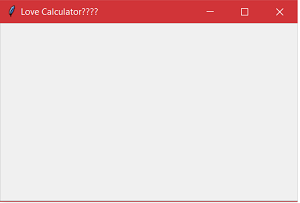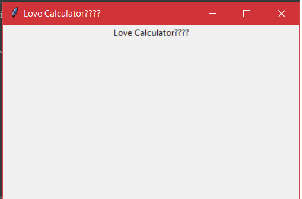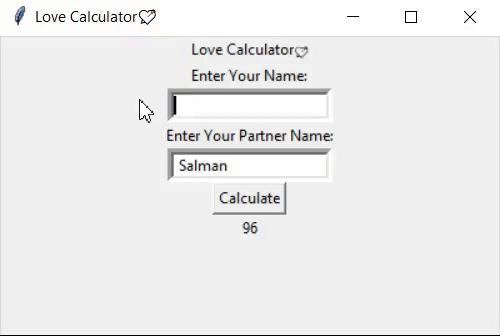Open in App
Not now

# Love Calculator GUI Application in Python

• Difficulty Level : Medium
• Last Updated : 13 Jul, 2022

In this article, we are going to know how to make a GUI love calculator application using python.

Prerequisite: Tkinter application, Python Random function.

There are multiple ways in python to build a GUI(Graphical User Interface).  Among all of those, the most commonly used one is Tkinter. It’s the fastest and easiest way to make GUI applications with Python.  To use Tkinter, we have to import the Tkinter module and create a container and perform the required operations.

In this article Let’s build a GUI-based Love Calculator, using the Python Tkinter module. In this GUI application user has to give input his/her name and his/her partner’s name. And the GUI application will show the Love percentage between the partners.

This GUI application Looks Like this:## Implementation

Step 1: Import the Tkinter package and all of its modules.

Step 2: Import Random Module. It’s an in-built module of Python which is used to generate random numbers.

Step 3: Create a Root window and set a proper title of the window using title() and set dimension using geometry(). Then Use mainloop() at the end to create an endless loop.

## Python3

 `# import tkinter ` `from` `tkinter ``import` `*` `# import random module ` `import` `random ` `# Creating GUI window ` `root ``=` `Tk() ` `# Defining the container size, width=400, height=240 ` `root.geometry(``'400x240'``) ` `root.title(``'Love Calculator????'``)  ``# Title of the container ` ` `  ` `  `# Starting the GUI ` `root.mainloop() `

Output:Step 4: Now create a function (calculate_love())which will generate two digits random numbers using random().

## Python3

 `# import tkinter ` `from` `tkinter ``import` `*` `# import random module ` `import` `random ` `# Creating GUI window ` `root ``=` `Tk() ` `# Defining the container size, width=400, height=240 ` `root.geometry(``'400x240'``) ` `root.title(``'Love Calculator????'``)  ``# Title of the container ` ` `  `# Function to calculate love percentage between the user and partner ` `def` `calculate_love(): ` `    ``# value will contain digits between 0-9 ` `    ``st ``=` `'0123456789'` `    ``result``=``0` `    ``# result will be in double digits ` `    ``digit ``=` `2` `    ``temp ``=` `"".join(random.sample(st, digit)) ` `    ``result.config(text``=``temp) ` ` `  ` `  `# Starting the GUI ` `root.mainloop() `

Step 5: We’ll add a label or heading using the Label Class and change its text configuration as desired. Here pack is a geometry manager which packs all the widgets one after the other in the root window. It’s much easier to use than grid manager but its uses are somewhat limited.

## Python3

 `# import tkinter ` `from` `tkinter ``import` `*` `# import random module ` `import` `random ` `# Creating GUI window ` `root ``=` `Tk() ` `# Defining the container size, width=400, height=240 ` `root.geometry(``'400x240'``) ` `# Title of the container ` `root.title(``'Love Calculator????'``) ` ` `  `# Function to calculate love percentage between the user ans partner ` `def` `calculate_love(): ` `    ``# value will contain digits between 0-9 ` `    ``st ``=` `'0123456789'` `    ``result``=``0` `    ``# result will be in double digits ` `    ``digit ``=` `2` `    ``temp ``=` `"".join(random.sample(st, digit)) ` `    ``result.config(text``=``temp) ` ` `  `# Heading on Top ` `heading ``=` `Label(root, text``=``'Love Calculator????'``) ` `heading.pack() ` ` `  `# Starting the GUI ` `root.mainloop() `

Output:Step 6: Now create two slots for the user and his/her partner to display their names as input using Label class. The label is a widget of Tkinter used to display boxes where images or text can be placed.

## Python3

 `# import everything from tkinter module ` `# import tkinter ` `from` `tkinter ``import` `*` `# import random module ` `import` `random ` `# Creating GUI window ` `root ``=` `Tk() ` `# Defining the container size, width=400, height=240 ` `root.geometry(``'400x240'``) ` `root.title(``'Love Calculator????'``)  ``# Title of the container ` ` `  `# Function to calculate love percentage between the user ans partner ` `def` `calculate_love(): ` `    ``st ``=` `'0123456789'`  `# value will contain digits between 0-9 ` `    ``digit ``=` `2`  `# result will be in double digits ` `    ``temp ``=` `"".join(random.sample(st, digit))  ``# result ` `    ``result.config(text``=``temp) ` ` `  `# Heading on Top ` `heading ``=` `Label(root, text``=``'Love Calculator????'``) ` `heading.pack() ` ` `  `# Slot/input for the first name ` `slot1 ``=` `Label(root, text``=``"Enter Your Name:"``) ` `slot1.pack() ` `name1 ``=` `Entry(root, border``=``5``) ` `name1.pack() ` ` `  `# Slot/input for the partner name ` `slot2 ``=` `Label(root, text``=``"Enter Your Partner Name:"``) ` `slot2.pack() ` `name2 ``=` `Entry(root, border``=``5``) ` `name2.pack() ` ` `  `# Starting the GUI ` `root.mainloop() `

Output:Step 7: Now we will add a button on the root window and will set its properties like height and width. Also, we set a command that is the function we created before to generate a random number. So when the button will be clicked it will redirect to the calculate_love() function which will generate two digits random number.

## Python3

 `# Python Tkinter GUI based "LOVE CALCULATOR" ` ` `  `# import tkinter ` `from` `tkinter ``import` `*` `# import random module ` `import` `random ` `# Creating GUI window ` `root ``=` `Tk() ` `# Defining the container size, width=400, height=240 ` `root.geometry(``'400x240'``) ` `# Title of the container ` `root.title(``'Love Calculator????'``) ` ` `  `# Function to calculate love percentage ` `# between the user ans partner ` ` `  ` `  `def` `calculate_love(): ` `    ``# value will contain digits between 0-9 ` `    ``st ``=` `'0123456789'` `    ``# result will be in double digits ` `    ``digit ``=` `2` `    ``temp ``=` `"".join(random.sample(st, digit)) ` `    ``result.config(text``=``temp) ` ` `  ` `  `# Heading on Top ` `heading ``=` `Label(root, text``=``'Love Calculator????'``) ` `heading.pack() ` ` `  `# Slot/input for the first name ` `slot1 ``=` `Label(root, text``=``"Enter Your Name:"``) ` `slot1.pack() ` `name1 ``=` `Entry(root, border``=``5``) ` `name1.pack() ` ` `  `# Slot/input for the partner name ` `slot2 ``=` `Label(root, text``=``"Enter Your Partner Name:"``) ` `slot2.pack() ` `name2 ``=` `Entry(root, border``=``5``) ` `name2.pack() ` ` `  `# create a Button and place at a particular ` `# location inside the root window . ` `# when user press the button, calculate_love function ` `# affiliated to that button is executed . ` `# 'text' used to define text on button and ` `# height and width defines those properties of button ` `bt ``=` `Button(root, text``=``"Calculate"``, height``=``1``, ` `            ``width``=``7``, command``=``calculate_love) ` `bt.pack() ` ` `  `# Text on result slot ` `result ``=` `Label(root, text``=``'Love Percentage between both of You:'``) ` `result.pack() ` ` `  `# Starting the GUI ` `root.mainloop() `

Output:Final Output:My Personal Notes arrow_drop_up
Related Articles